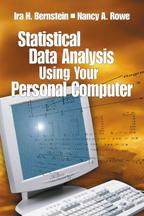# Statistical Data Analysis Using Your Personal Computer

What should you see when you are analyzing real data using one of the major statistical packages, such as SPSS, SAS or Microsoft Excel? This book will show you, and will walk you through the output from a variety of statistical outcomes, such as data reflecting a single common factor. Through the use of actual demonstrations, the authors supply readers with the computer programs necessary to simulate data sets with the statistical properties (usually multivariate) that are often assumed of real data. The reader is then shown how to analyze these data sets and how to interpret the results. The book begins with a general introduction to doing research and tips for using the three statistical packages. The authors next explore how to create data structures and perform univariate, bivariate, and multivariate simulations. They then show how to use the simulations to understand common statistical algorithms and their outputs when doing a basic correlation analysis, exploratory factor analysis, confirmatory factor analysis, multidimensional scaling, multiple regression, discriminate analysis, classification analysis and MANOVA. Throughout the book, the authors provide the reader with helpful guides, such as: *Hint boxes to give readers tips for executing particular techniques using the statistical software packages. *Steps that show each stage of a procedure, such as importing an Excel file into SAS. *Problems end each chapter so the reader can practice the techniques described. *Web Site with the SAS and SPSS programs and sample data.

•••